Ask your WordPress questions! Pay money and get answers fast!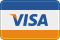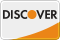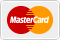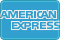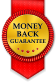# Passing category ID variable to determine expiration WordPress

• SOLVED

Hello,
What I'm trying to do here is catch the post ID and pass it into a function that will determine the expiration date of a post.

The code below belongs to a PHP page that is processing a form on a classifieds page. The form passes the values to this file and it turns the values into custom fields, etc.

Earlier in the code the cat is defined like this:

``` \$post_cat = (int)cp_filter(\$_POST['cat']); \$post_cat_array = array("\$post_cat"); ```

Down the page a bit more is where I <em>believe</em> I need help. Where I have the "in_category" function, I think I need something like "if \$post_cat = 30 {do this}". I believe this should be possible since the category is being defined previously.

``` \$post_id = wp_insert_post( array( 'post_author' => \$user_id, 'post_title' => \$post_title, 'post_content' => \$description, 'post_category' => \$post_cat_array, 'post_status' => \$post_status, 'tags_input' => \$post_tags ) ); //the issue lies in this 'custom' function if ( in_category( 4 )) { \$ad_length = 30; \$expires = date('m/d/Y G:i:s', strtotime("+" . \$ad_length . " days")); } elseif ( in_category( 14 )) { \$ad_length = 365; \$expires = date('m/d/Y G:i:s', strtotime("+" . \$ad_length . " days")); } //end of that custom function add_post_meta(\$post_id, 'location', \$location, true); add_post_meta(\$post_id, 'city', \$city, true); add_post_meta(\$post_id, 'state', \$state, true); add_post_meta(\$post_id, 'neighborhood', \$neighborhood, true); add_post_meta(\$post_id, 'zip', \$zip, true); add_post_meta(\$post_id, 'zip2', \$zip2, true); add_post_meta(\$post_id, 'zip3', \$zip3, true); add_post_meta(\$post_id, 'price', \$price, true); add_post_meta(\$post_id, 'name', \$name, true); add_post_meta(\$post_id, 'email', \$email, true); add_post_meta(\$post_id, 'phone', \$phone, true); add_post_meta(\$post_id, 'images', \$images, true); add_post_meta(\$post_id, 'expires', \$expires, true); add_post_meta(\$post_id, 'cp_adURL', \$cp_adURL, true); ```

If there is an easier way to go about this, that would also be welcomed!

Thanks!
Mike

2010-05-13

```if ( \$post_cat == 4 ) { \$ad_length = 30; \$expires = date('m/d/Y G:i:s', strtotime("+" . \$ad_length . " days")); } elseif ( \$post_cat == 14 ) { \$ad_length = 365; \$expires = date('m/d/Y G:i:s', strtotime("+" . \$ad_length . " days")); }```

``` //do_action( "added_{\$meta_type}_meta", \$wpdb->insert_id, \$object_id, \$meta_key, \$meta_value ); add_action('added_post_meta', 'added_post_meta_filter',1,4); function added_post_meta_filter(\$postid,\$objectid,\$mk,\$vl){ if (\$mk == 'exiers'){ //here is should be get post categories, // is post in categories, // is yeas filter data // and direct update thought wpdb } }```